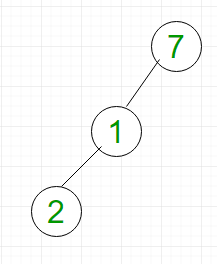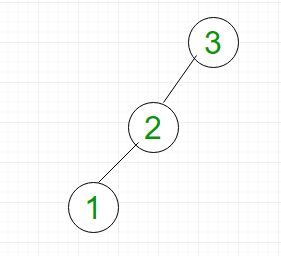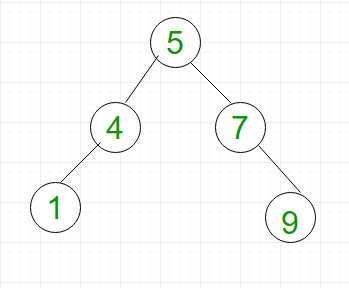# Complexity of different operations in Binary tree, Binary Search Tree and AVL tree

In this article, we will discuss complexity of different operations in binary trees including BST and AVL trees. Before understanding this article, you should have basic idea about: Binary Tree, Binary Search Tree and AVL Tree.

The main operations in binary tree are: search, insert and delete. We will see the worst case time complexity of these operations in binary trees.

Binary Tree –
In a binary tree, a node can have maximum two children. Consider the left skewed binary tree shown in Figure 1.• Searching: For searching element 2, we have to traverse all elements (assuming we do breadth first traversal). Therefore, searching in binary tree has worst case complexity of O(n).
• Insertion: For inserting element as left child of 2, we have to traverse all elements. Therefore, insertion in binary tree has worst case complexity of O(n).
• Deletion: For deletion of element 2, we have to traverse all elements to find 2 (assuming we do breadth first traversal). Therefore, deletion in binary tree has worst case complexity of O(n).

Binary Search Tree (BST) –
BST is a special type of binary tree in which left child of a node has value less than the parent and right child has value greater than parent. Consider the left skewed BST shown in Figure 2.• Searching: For searching element 1, we have to traverse all elements (in order 3, 2, 1). Therefore, searching in binary search tree has worst case complexity of O(n). In general, time complexity is O(h) where h is height of BST.
• Insertion: For inserting element 0, it must be inserted as left child of 1. Therefore, we need to traverse all elements (in order 3, 2, 1) to insert 0 which has worst case complexity of O(n). In general, time complexity is O(h).
• Deletion: For deletion of element 1, we have to traverse all elements to find 1 (in order 3, 2, 1). Therefore, deletion in binary tree has worst case complexity of O(n). In general, time complexity is O(h).

AVL/ Height Balanced Tree –
AVL tree is binary search tree with additional property that difference between height of left sub-tree and right sub-tree of any node can’t be more than 1. For example, BST shown in Figure 2 is not AVL as difference between left sub-tree and right sub-tree of node 3 is 2. However, BST shown in Figure 3 is AVL tree.• Searching: For searching element 1, we have to traverse elements (in order 5, 4, 1) = 3 = log2n. Therefore, searching in AVL tree has worst case complexity of O(log2n).
• Insertion: For inserting element 12, it must be inserted as right child of 9. Therefore, we need to traverse elements (in order 5, 7, 9) to insert 12 which has worst case complexity of O(log2n).
• Deletion: For deletion of element 9, we have to traverse elements to find 9 (in order 5, 7, 9). Therefore, deletion in binary tree has worst case complexity of O(log2n).

We will discuss questions based on complexities of binary tree operations.

Que-1. What is the worst case time complexity for search, insert and delete operations in a general Binary Search Tree?
(A) O(n) for all
(B) O(Logn) for all
(C) O(Logn) for search and insert, and O(n) for delete
(D) O(Logn) for search, and O(n) for insert and delete

Solution: As discussed, all operations in BST have worst case time complexity of O(n). So, the correct option is (A).

Que-2. What are the worst case time complexities of searching in binary tree, BST and AVL tree respectively?
(A) O(n) for all
(B) O(Logn) for all
(C) O(n) for binary tree, and O(Logn) for others
(D) O(n) for binary tree and BST, and O(Logn) for AVL

Solution: As discussed, search operation in binary tree and BST have worst case time complexity of O(n). However, AVL tree has worst case time complexity of O(logn). So, the correct option is (D).My Personal Notes arrow_drop_up

This article is contributed by Sonal Tuteja. If you like GeeksforGeeks and would like to contribute, you can also write an article using contribute.geeksforgeeks.org or mail your article to contribute@geeksforgeeks.org. See your article appearing on the GeeksforGeeks main page and help other Geeks.

Please Improve this article if you find anything incorrect by clicking on the "Improve Article" button below.

Improved By : nitinshivanandmesta

Article Tags :
Practice Tags :

17

Please write to us at contribute@geeksforgeeks.org to report any issue with the above content.